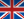Une voie, plusieurs choix
Informatique et Mathématiques appliquées# Partial differential equations and finite difference method - 4MMMEDP6

A+Augmenter la taille du texteA-Réduire la taille du texteImprimer le documentEnvoyer cette page par mail cet article Facebook Twitter Linked In
• #### Number of hours

• Lectures : 16.5
• Tutorials : 16.5
• Laboratory works : -
• Projects : -
• Internship : -
• Written tests : -
ECTS : 3.0
• Officials : Eric BLAYO

### Goals

Numerical solutions of Partial Differential Equations are at the center of computational science. The object of this course is to present the main standard PDEs and to give the principles of the finite difference method. Every chapter contains the continuous problem and several discrete algorithms.
The course is made of lectures, exercise sessions, and practical Python demos.

Content

• Introduction : Mathematical modelling with PDEs.
I - Generalities on PDEs
II - The finite difference method.
Examples. Consistency, stability and convergence.
III - Elliptic equations : Laplace and Poisson equations, maximum principle, mean value property, n-D finite difference schemes
IV - Parabolic equations : Diffusion phenomena.
Analytic solutions, Fourier's method.
Finite difference schemes (forward, backward, splitting, non linear case). Stability analysis.
Multi-D case.
V Hyperbolic equations. Propagation phenomena.
Transport equation, characteristics, domain of dependence,
Finite difference schemes.
Waves equation
Non linear case: Burgers. Characteristics, discontinuous solutions.

Prerequisites

Mathematical analysis (normed spaces, elementary Fourier analysis), linear algebra, basic numerical methods.

Tests

An exam at the end of the term (E).

N1=E1
N2=E2

The exam is given in english onlyCalendar

The course exists in the following branches:

• Curriculum - Math. Modelling, Image & Simulation - Semester 7 (this course is given in english only)
see the course schedule for 2020-2021

Course ID : 4MMMEDP6
Course language(s):The course is attached to the following structures:

You can find this course among all other courses.

Bibliography

G. Allaire. Analyse numerique et optimisation. Editions de l’Ecole Polytechnique, 2006.
L. Evans. Partial Differential Equations. Graduate Studies in Mathematics, Vol 19, American Math. Society, 1998.
R. Leveque. Finite difference methods for ordinary and partial differential equations. SIAM, 2007.
B. Mohammadi, JH Saiac. Pratique de l’analyse numerique. Dunod, 2003

A+Augmenter la taille du texteA-Réduire la taille du texteImprimer le documentEnvoyer cette page par mail cet article Facebook Twitter Linked In

Date of update January 15, 2017École nationale supérieure d'informatique et de mathématiques appliquées
681, rue de la passerelle - Domaine universitaire - BP 72
38402 SAINT MARTIN D'HERES